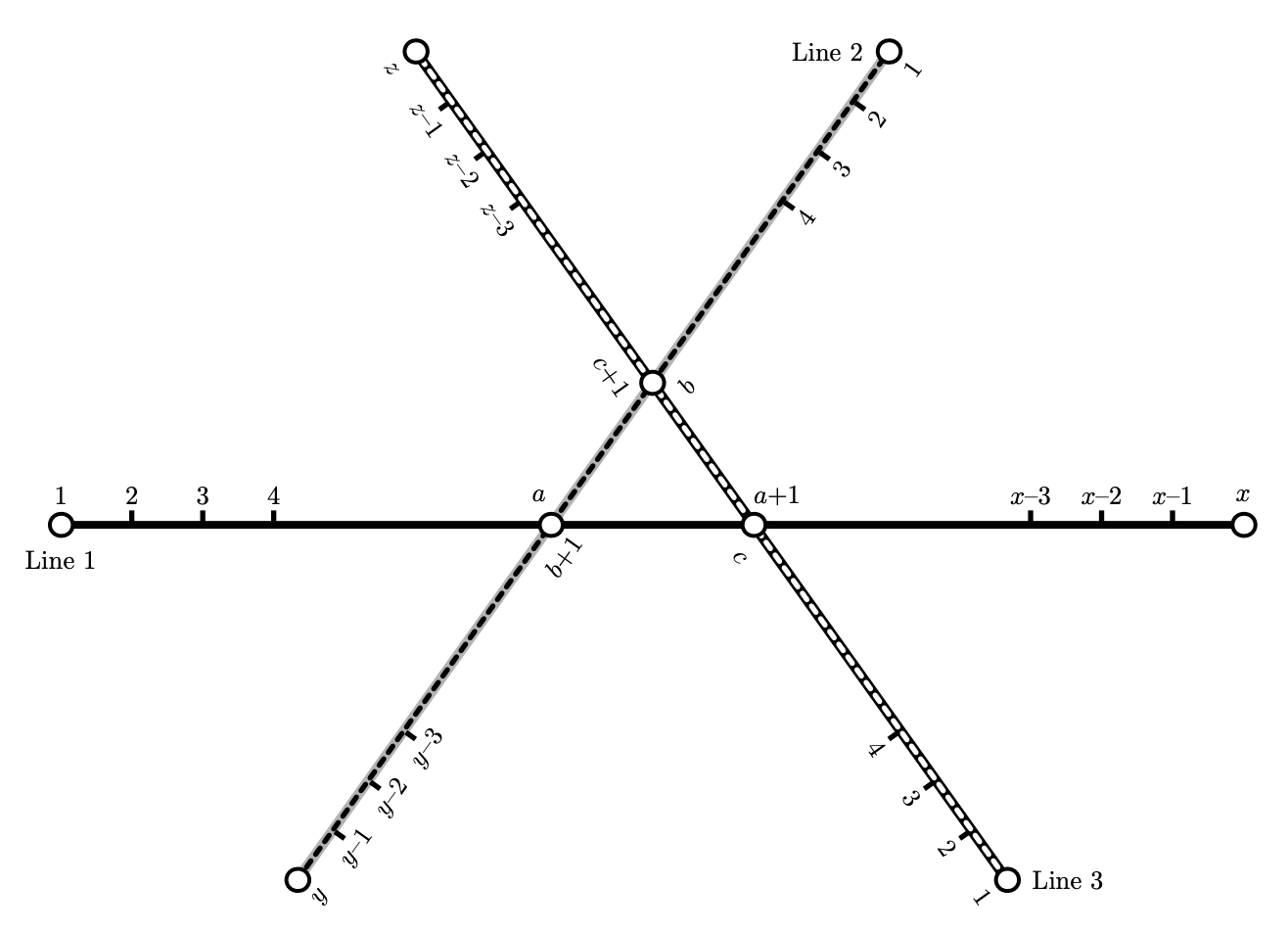시간 제한 메모리 제한 제출 정답 맞은 사람 정답 비율
1 초 512 MB 0 0 0 0.000%

## 문제

Kekopolis has three metro lines, they intersect near the city center, forming a triangle of three tunnels between adjacent stations. The first line has $x$ stations, the second line has $y$ stations, the third line has $z$ stations. Stations at each line are numbered consequently starting from $1$.

Stations where the lines intersect allow passengers to change between lines. It is possible to change between station $a$ of the first line and station $b+1$ of the second line, station $a+1$ of the first line and station $c$ of the third line, and station $b$ of the second line and station $c+1$ of the third line.The lines have different speed of trains. The train at the first line travels between adjacent stations in $t_1$ seconds, the train at the second line does it in $t_2$ seconds, the train at the third line does it in $t_3$ seconds. It takes $d$ seconds to change between lines. Let us ignore the time to enter or leave the station or the train, and the time the train stops at the station.

Dima needs to get to Kekopolis Programming Contest. He starts his way at home that is located near station $i$ of the $k$-th line, and needs to get to Kekopolis University that is located near station $j$ of the $l$-th line. Dima overslept as usually, so he is getting late. Dima wants to get to his destination as soon as possible.

Find the minimum time Dima needs to get from his home the the university. Dima doen't enter nor exists metro at exchange station.

## 입력

The first line of input contains three integers $x$, $y$ and $z$ ($2 \le x, y, z \le 10^9$).

The second line contains three integers $a$, $b$ and $c$ ($1 \le a < x$, $1 \le b < y$, $1 \le c < z$).

The third line contains four integers $t_1$, $t_2$, $t_3$ and $d$ ($1 \le t_1, t_2, t_3, d \le 10^9$).

The fourth line contains four integers $k$, $i$, $l$ and $j$ ($1 \le k, l \le 3$, it is guaranteed that the $k$-th line has station $i$, the $l$-th line has station $j$, the starting station is different from the destination station, in particular they are not the two stations at the same interchange).

## 출력

Output one integer --- the minimum time needed to travel from the starting station to the destination station.

## 예제 입력 1

4 4 4
2 2 2
1 1 1 1
1 1 2 1


## 예제 출력 1

4


## 예제 입력 2

4 4 4
2 2 2
1 10 1 1
1 1 3 4


## 예제 출력 2

5


## 예제 입력 3

4 4 4
2 2 2
1 1 1 1
1 1 1 4


## 예제 출력 3

3